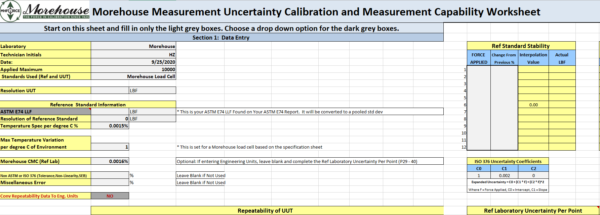# Customer Support

Morehouse has a collection of tools, videos & documentation to assist customers in optimizing their calibration & testing products. Use the filter on the left to assist in finding the specific support item needed.
Narrow The Choices

# #1 CMC Calculation Made Easy Tool for Force Uncertainty

Are you having problems figuring out all of the requirements to calculate a CMC for force uncertainty or torque uncertainty? This excel sheet provides a template to calculate CMCs (force uncertainty) with explanations of everything required to pass an ISO/IEC 17025 audit.Morehouse Free Force Uncertainty Spreadsheet to Calculate Calibration and Measurement Capability Uncertainty

# Measurement Risk and TUR Calculator Tool - 1 Simple to use Tool

Decision Rules can be difficult to understand, and this worksheet will help simplify things by focusing on measurement risk. The Morehouse Measurement risk TUR calculator tool will cure those measurement headaches allowing anyone to calculate TUR properly and Measurement Risk Correctly. Use this tool to input parameters such as accuracy requirement, resolution, measurement uncertainty, TUR, and repeatability with other error sources.

The TUR calculator will calculate how good the reference standard uncertainty needs to be to maintain test uncertainty (TUR) ratios and will allow the end-user to input their own risk tolerances. The TUR Calculator can be found here.

# Easy to Use Mass to Force Conversion Tool

Mass to Force Conversion tool solves issues with results not agreeing because the same units are not being used. This mass to force spreadsheet tool was created to help convert mass to force and mass to force.

# ASTM E74 Load Cell Selection Guide

ASTM E74 Load Cell Selection Guide

Need to know what 0.005 %, and 0.01 % of full scale means when calibrating to a specified standard such as ASTM E74? This spreadsheet breaks these numbers down into a % of reading at various force points throughout the calibration range. The ASTM E74 load cell selection guide is a free excel spreadsheet designed to simplify the selection of ASTM E74 load cells. Click here to download the load cells selection guide.

# Morehouse Force Software Verification

Morehouse force software verification excel sheet. The excel sheet verifies the force software from Morehouse. The sheet includes formulas and screenshots to prove the Morehouse force calibration does what it should. Download our force verification sheet force software verification.

# Easy to Use Tool for Calculating Expanded Uncertainty Per Point at the time of Calibration

Want to know how we are calculating expanded uncertainty on force and torque certificates? This spreadsheet has all of the formulas that comply with ILAC-P14:09/2020 ILAC Policy for
Measurement Uncertainty in Calibration at the time of calibration. Calculating Expanded Uncertainty is drastically simplified and easily explained with this tool

# ASTM E74 Coefficient Calculator

The ASTM E74 Coefficient Calculator is free software from Morehouse to help calculate higher-order coefficients per ASTM E74 and ISO 376.  ASTM E74 Coefficient Calculator contains macros because it uses visual basic coding to generate the coefficients.

# Easy to Use Resolution, TUR, and Measurement Risk Calculator

Measurement Risk Calculator presented at A2LA tech forum and NCSLI, this easy-to-use Measurement Risk Calculator tool allows one to determine the effect of the resolution of an instrument on the overall measurement uncertainty and risk. One can use this tool to analyze the effects on TUR and measurement risk as well.

# Method B Interpolated Zero Calculator

Interpolated Zero Calculator - Use this free calculator to easily interpolate the ending zero throughout your dataset. Compliant with ASTM E74 Method B and ISO 376 for ending zero reduction. Download the Interpolated Zero Calculator tool by clicking here

# Probability of False Accept (PFA) Worksheet

Probability of False Accept - Free Excel Worksheet. Decision Rules can be difficult to understand, but this worksheet will help. Use this tool to input parameters such as accuracy requirement, resolution, measurement uncertainty, and repeatability with other error sources. It will help make decisions on equipment, such as: Should I buy a device with better resolution? Do I need my calibration provider to have better measurement uncertainty than what I am getting? It also provides different Methods, using different decision rules (Method 5 and 6). The PFA calculator contains macros.

Spreadsheet to print Load Cells Load Tables. When working to ASTM E4, sometimes it's easier to have printed tables that have set to force points.

# Proving Rings Load Tables

###### Proving Rings Load Tables

Proving Ring Load Tables spreadsheet to print load tables for Proving Rings. This is used for ASTM E74 calibrations where the end-user does not want to use a computer and wants to print tables to interpolate force points. The proving ring load tables sheet can be downloaded here.

# Proving Rings Used as Standards in Morehouse UCM

Proving Rings Used as Standards is a Free excel sheet to correct for temperature and tare weight when using Morehouse Proving Rings. Calibration load points for Proving Rings used as standards in a Morehouse UCM. Click here to download Proving Rings Used as Standards excel sheet.

# Morehouse A and B Coefficients for Load Cells

A and B Coefficients for Load Cells. This free Load table generator for Morehouse A and B Coefficients is found on calibration certificates. This useful tool allows you to print load tables

# Force Conversion LBF to KGF and N

Force Conversion Spreadsheet to Convert LBF to KGF and N for Calibration load points in compression and tension.

Morehouse Instrument Company was founded in 1920 and provides calibration services and products that help lower measurement risk.

1742 Sixth Ave.
York, PA 17403
USA

+1 (717) 843-0081

info@mhforce.com

Top